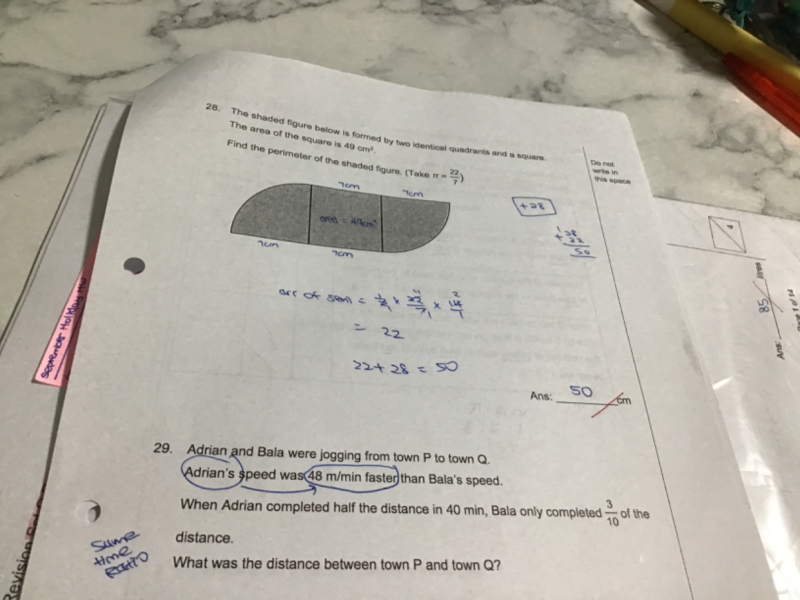# Questionpls help with qn 29, thanks

P —————————————————- Q

<————————–d———————–>

Bala speed = u

Adrian speed = u + 48

In 40 mins,  using  speed x time = distance,

40u = (3/10)d

40(u+48) = (5/10)d  => 40u + 1920 = (5/10)d

Difference in distance between Adrian and Bala = (2/10)d = 1920  => d = 9600

Hence the distance between P and Q is 9600m

0 Replies 1 Like ✔Accepted Answer